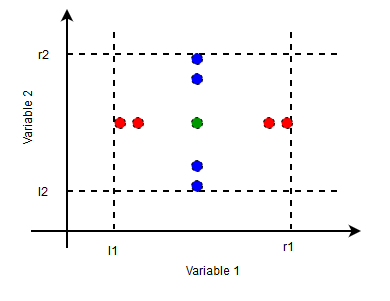# Boundary Value Analysis – Triangle Problem

• Last Updated : 11 Jun, 2021

Boundary Value Analysis (BVA) is a black box software testing technique where test cases are designed using boundary values. BVA is based on the single fault assumption, also known as critical fault assumption which states that failures are rarely the product of two or more simultaneous faults. Hence while designing the test cases for BVA we keep all but one variable to the nominal value and allowing the remaining variable to take the extreme value.

Test Case Design for BVA:
While designing the test cases for BVA first we determine the number of input variables in the problem. For each input variable, we then determine the range of values it can take. Then we determine the extreme values and nominal value for each input variable.

Attention reader! Don’t stop learning now. Get hold of all the important CS Theory concepts for SDE interviews with the CS Theory Course at a student-friendly price and become industry ready.

Consider an input variable t taking values in the range [l, r].Extreme values for t are –```t = l
t = l+1
t = r-1
t = r ```

The nominal value for the variable can be any value in the range (l, r).
In most of the BVA implementations, it is taken as the middle value of the range (r+l)/2.
The figure on the right shows the nominal and extreme values for boundary value of analysis of a two variable problem.

Under the single fault assumption, the total number of test cases in BVA for a problem with n inputs is 4n+1.
The 4n cases correspond to the test cases with the four extreme values of each variable keeping the other n-1 variable at nominal value. The one additional case is where all variables are held at a nominal value.

One of the common problem for Test Case Design using BVA is the Triangle Problem that is discussed below –

Triangle Problem accepts three integers – a, b, c as three sides of the triangle .We also define a range for the sides of the triangle as [l, r] where l>0. It returns the type of triangle (Scalene, Isosceles, Equilateral, Not a Triangle) formed by a, b, c.

For a, b, c to form a triangle the following conditions should be satisfied –

```a < b+c
b < a+c
c < a+b ```

If any of these conditions is violated output is Not a Triangle.

• For Equilateral Triangle all the sides are equal.
• For Isosceles Triangle exactly one pair of sides is equal.
• For Scalene Triangle all the sides are different.

The table shows the Test Cases Design for the Triangle Problem.The range value [l, r] is taken as [1, 100] and nominal value is taken as 50.

The total test cases is,

`4n+1 = 4*3+1 = 13 `

My Personal Notes arrow_drop_up